cancel
Showing results for
Did you mean:

Fabric is Generally Available. Browse Fabric Presentations. Work towards your Fabric certification with the Cloud Skills Challenge.## How to get the value in a column based on condition of another column in Power Query

Scenario:

In Power BI we can easily get a value with a specific condition by DAX. Which, however, will be more complicated for M language beginners in Power Query. This blog will introduce you how to get the value in a column based on condition of another column in Power Query.

Example: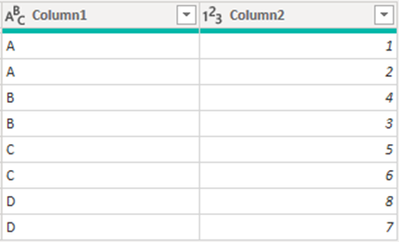Expected result: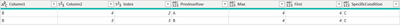• Get the value of previous row in Column1.
• For the same value in Column1, pick its maximum value in Column2.
• For the same value in Column1, pick its first value in Column2.
• Pick the first value in Column1 whose corresponding value in Column2 is greater than 5.
• Extract rows with the value B in column 1.

Solution:

1. Add an index column in the table.1. Add a custom column or use code directly in other M expression that requires this result.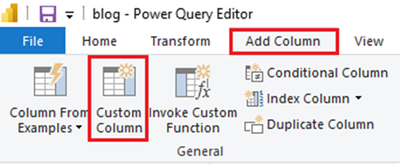Scenario 1: Get the value of previous row in Column1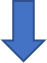code interpretation:

(x)=>x[Index]: This is a function in M language. The function-expression is:parameter-list ) function-return-type => function-body, parameter-list and return-type are optional. Here it will return a table containing only the rows that match a condition, similar with the expression “FILTER(ALL(‘Table’), <filter>)” in DAX.

Table.SelectRows(table, condition)[Column1]{0}: The Table.SelectRows function will return a filtered table, next add a column which will return a corresponding filtered list. Add an expression {number} after a list will return a particular record, the number in the symbol {} represents the value of the row number in the list minus 1. So here in the sample, {0} will return the first row in the list. As there’s only one row filtered based on the condition “[Index]=[Index]-1”, the previous value in the column return.

Here are more extended scenarios and solutions:

Scenario 2: For the same value in Column1, pick its maximum value in Column2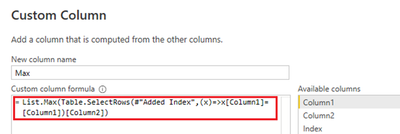Scenario 3: For the same value in Column1, pick its first value in Column2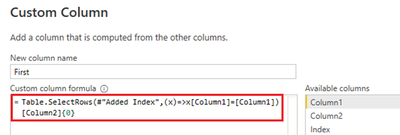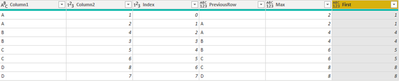Scenario 4: Pick the first value in Column1 whose corresponding value in Column2 is greater than 5Scenario 5: Extract rows with the value B in column 1

In this scenario, open a new blank query and copy  the below code.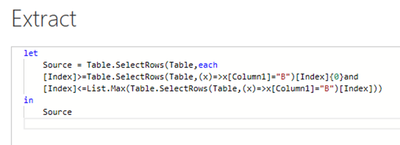Whole M syntax:

Scenario 1 to Scenario 4:

``````let

Source = Table.FromRows(Json.Document(Binary.Decompress(Binary.FromText("i45WclTSUTJUitWBsIzALCcgywTOMgaznIEsUzjLDMxyAbIs4CxzpdhYAA==", BinaryEncoding.Base64), Compression.Deflate)), let _t = ((type nullable text) meta [Serialized.Text = true]) in type table [Column1 = _t, Column2 = _t]),

#"Changed Type" = Table.TransformColumnTypes(Source,{{"Column1", type text}, {"Column2", Int64.Type}}),

in

Scenario 5:

``````let

Source = Table.SelectRows(Table,each [Index]>=Table.SelectRows(Table,(x)=>x[Column1]="B")[Index]{0}and [Index]<=List.Max(Table.SelectRows(Table,(x)=>x[Column1]="B")[Index]))

in

Source``````

Author: Yan Jiang

Reviewer: Kerry Wang & Ula Huang